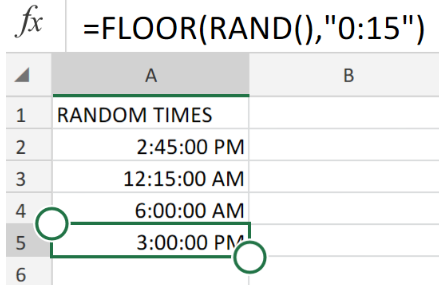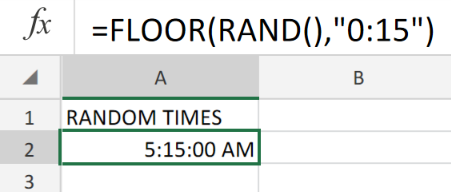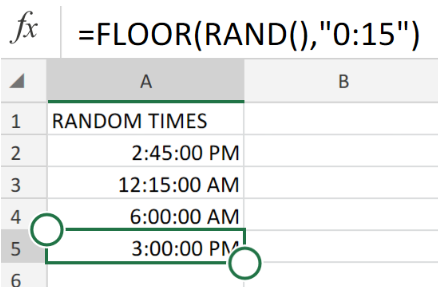Get instant live expert help with Excel or Google Sheets“My Excelchat expert helped me in less than 20 minutes, saving me what would have been 5 hours of work!”

#### Post your problem and you'll get expert help in seconds

Your message must be at least 40 characters
Our professional experts are available now. Your privacy is guaranteed.

# How to Get Random Times at Specific Intervals in ExcelRead time: 20 minutesFigure 1. Random Times at Specific Intervals in Excel.

Let’s say we have to come up with random times within specific intervals in Excel, we must utilize the RAND and FLOOR functions.

## Generic Formula

`=FLOOR(RAND(),"0:15")`

The Excel RAND and FLOOR Formula requires no input parameters to generate random decimal numbers from time intervals between 0 and 1.

## How to use the RAND and FLOOR functions in Excel.

Simple numbers are used to define Excel dates, Where 1 = 1 day.

Therefore, we can simply the decimal value of time by 1 to obtain a value which corresponds to time as Excel sees it.

Follow the 2 simple steps discussed below to observe how we apply the RAND and FLOOR functions.

1. In our worksheet example below the RAND and FLOOR formula we have entered into cell A2 is as follows;

`=FLOOR(RAND(),"0:15")`Figure 2. Random Times at Specific Intervals in Excel.

In our worksheet example above, RAND() generates a decimal number between 1 and 0, then the FLOOR Function proceeds to round that decimal number down to the nearest interval which are then expressed as recognized time intervals.

1. Enter the RAND and FLOOR formula in cell A2 above into a few other cells in the RANDOM TIMES column to achieve other results.Figure 3. Random Times at Specific Intervals in Excel.

## Note

Each time we enter the RAND and FLOOR formula, Excel will generate a random time within 15-minute intervals.

## Instant Connection to an Expert through our Excelchat Service:

Our live Excelchat Service is here for you. We have Excel Experts available 24/7 to answer any Excel questions you may have. Guaranteed connection within 30 seconds and a customized solution for you within 20 minutes.

### Did this post not answer your question? Get a solution from connecting with the expert.Another blog reader asked this question today on Excelchat:
Related blogs## Subscribe to Excelchat.coAnother blog reader asked this question today on Excelchat: